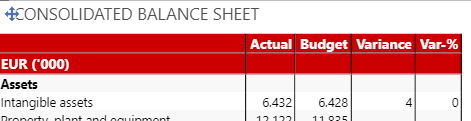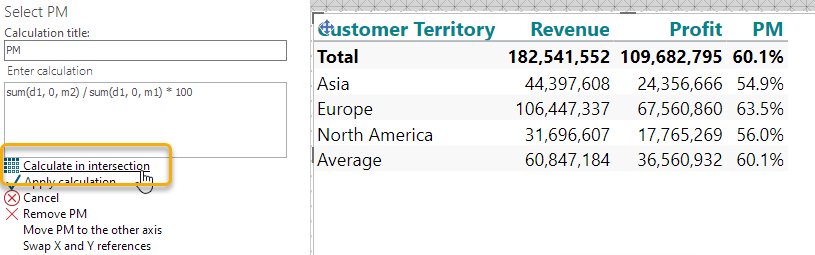# Conditional number format

Hi

I have made an advanced row calculation with a number of columns.

To my understanding I can only have one number format to a row calcultion.

Is it somehow possible to make a conditional number format (different format for the columns)

• I would like to have a percent format on the column "Var-%)• Would be interested in the solution, too :)

• Assuming 'Var - %' is a calculation, you need to select calculations then select 'Var - %' then you can select '# Format numbers' and apply formatting to only the desired columns.

• Thanks Daniel, but since my row "Intangible assets" is a row added in advanced calculations, this option is not possible.

• Hi Flemming,

You are correct that a calculation can only have one number format.

However, like Daniel, I presume that 'Var - %' is a calculated column and that you have used the 'Calculate in intersection' option to show the '0'...?

In that case, Daniel is correct. You should be able to go 'Calculations', select the 'Var - %' calculation and do the 'Calculate in intersection' trick to get the proper formatting. Se my screenshot where 'PM' is my calculated column and 'Average' is my calculated row:If this is not the case, then you need to elaborate a bit more on how your crosstab is designed.

BR / Ole

• Thank Ole.

It helped 90% ;-)

My only problem now is, that I have to use Calculate in intersection "the other way around" on two of the rows due to an if then else sentence in a column calculation.

But it's much better now ;-)